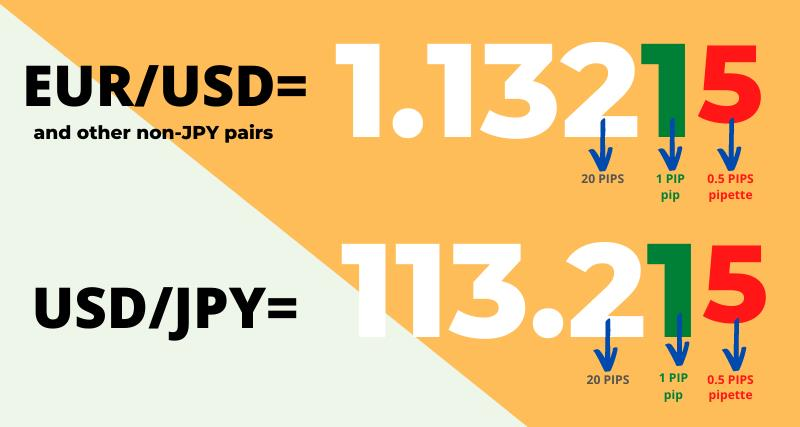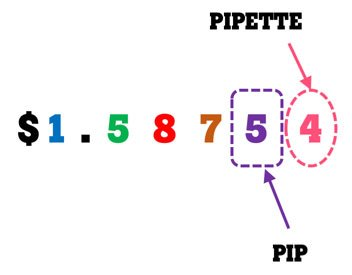# Understanding PIP value in Forex

## Understanding PIP value in ForexWhat is PIP value in Forex?

The pip value is the price attributed to a one-pip move in a forex trade – it is often used when referencing a position’s losses or gains. The meaning of pip value can vary between currencies, but as most major currency pairs are priced to four decimal places, a pip is usually equal to the fourth figure after the decimal point. In GBP/USD, for instance, 0.0001 is one pip.

Because pips are tiny in value, forex is traded in micro lots, mini lots and lots: 1000, 10,000 or 100,000 units of currency. Although the value of a pip isn’t that much, through leverage it can represent a significant exposure and can influence your open position considerably.

The pip value is defined by the currency pair being traded, the size of the trade and the exchange rate of the currency pair. To calculate pip value, divide one pip (usually 0.0001) by the current market value of the forex pair. Then, multiply that figure by your lot size, which is the number of base units that you are trading. This means that the value of a pip will be different between currency pairs, due to the variations in exchange rates. However, when the quote currency is the US dollar, the value of a pip is always the same – if the lot size is 100,000, the pip will equal \$10.

Usually you will not have to calculate the value of a pip yourself, as your forex broker or provider will do it for you, but it is a useful process to familiarise yourself with.

You can better understand it by looking at following pitctures...= = = = = = = = = =

What is PIPETTE?= = = = = = = = = =

1. FOR PAIRSS WITH USD AS THE COUNTER CURRENCY

where USD is wiritten last.
EURUSD
GBPUSD
NZDUSD
AUDUSD

Basically, the movement of a currency pair such as EUR/USD from 1.2000 to 1.2001 would represent a one pip rise in the exchange rate, so the pip size in EUR/USD is 0.0001. This one pip movement would equal a shift in value of \$0.10 on a micro lot of 1,000 euros, \$1 on a mini lot of 10,000 euros and \$10 for a full lot of 100,000 euros.  Those would be your pip values when trading in a U.S. dollar denominated account.

Therefore, to calculate the pip value for EUR/USD when the pip size is 0.0001, the spot rate 1.12034 and you are trading a position size of €100,000, you would plug that information into the formula shown in Step 3 above as follows:

(0.0001 / 1.20000) X €100,000 = €8.333333

Performing that calculation yields the pip value of €8.3333333. If you then want to calculate the U.S. dollar amount of this pip value, you must take the pip value of €8.925861791956013 and convert it into U.S. dollars by multiplying it by the EUR/USD exchange rate of 1.12034 as follows:

€8.333333 X 1.20000 \$/€ = \$101. FOR PAIRSS WITH USD AS THE BASE CURRENCY

Most other currency pairs have the U.S. dollar as the base currency, such as USD/JPY and USD/CAD, for example, and they have different pip values. To calculate the pip value where the USD is the base currency when trading in a U.S. dollar-denominated account, you need to divide the position size by the exchange rate.

For example, if the USD/CAD exchange rate is trading at 1.3000 and you have a \$100,000 position, then the pip value is one pip or 0.0001 x \$100,000 equals CAD\$10 since the Canadian dollar is the counter currency.

If you then wanted to convert that pip value into U.S. dollars, you would need to divide by the USD/CAD exchange rate of 1.3000 Canadian dollars per U.S. dollar, thereby yielding a USD pip value for that \$100,000 position of \$7.692307692307692.

3. COMPUTING PIP VALUES FOR CROSS CURRENCY PAIRS

To find the pip value of a currency pair where neither currency is the account currency, for example, when you are trading the EUR/GBP cross currency pair in a U.S. dollar-denominated account, you multiply the standard 10 pip value per full lot by the counter currency/account currency exchange rate, or GBP/USD in this example.

If the GBP/USD rate is 1.3000, that gives you a pip value of 10 x 1.3000 or \$13 for a EUR/GBP full lot position of 100,000 euros.

EASY WAY TO CALCUATE PIP VALUE

If your trading account with an online broker is funded with U.S. dollars, then any currency pair with the USD as the counter currency, such as EUR/USD, GBP/USD, AUD/USD or NZD/USD, will have a pip value of \$0.10 for a micro lot of 1,000 base currency units, \$1 for a mini lot of 10,000 base currency units or \$10 for a full lot of 100,000 base currency units.

To find the pip value when the USD is listed as the base currency, as in USD/JPY or USD/CAD, for an account denominated in U.S. dollars, divide the above-listed standard pip values per lot by the relevant exchange rate.

Thus, if you are trading a full lot of \$100,000 in the USD/CAD pair, then you divide the standard 10 pip value per full lot by the USD/CAD exchange rate. If the USD/CAD pair is trading at 1.3400, you will arrive at the correct pip value of 10 / 1.3400 = \$7.462686567164179 or \$7.46 per full lot when trading in an account denominated in U.S. dollars.

Successfully added Product to the Cart.
Failed.